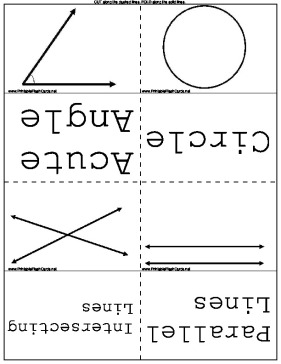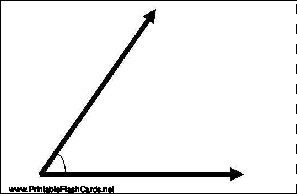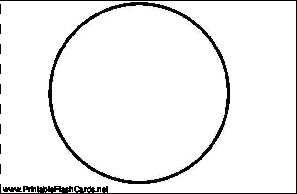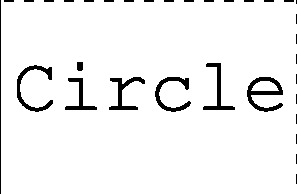Sample flash cards in this set:

Front:Back:This set includes the following cards:
(Image)Acute Angle
(Image)Circle
(Image)Intersecting Lines
(Image)Parallel Lines
(Image)Perpendicular Lines
(Image)Rectangle
(Image)Rhombus
(Image)Right Angle
(Image)Square
(Image)Straight Angle
(Image)Triangle
(Image)Obtuse Angle
(Image)Oval